# Learning How to Convert Fractions into DecimalsLast time we talked math we went over a classic algebra practice problem and learned how to solve for x. Today, in the latest edition of Math with Margo, converting fractions into decimals is a breeze!

In this simple tutorial, our resident math expert will walk you through an easy-to-follow example!

### How to convert fractions into decimals

Problem: How is the fraction ⅚ represented as a decimal?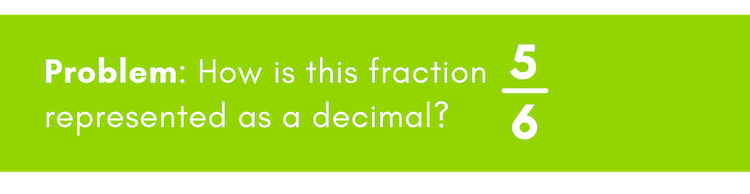#### Step One: Write out the problem

The fraction bar is also known as division, so this can be read as 5 divided by 6.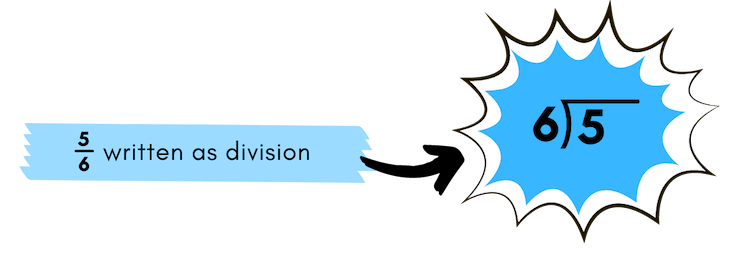#### Step Two: Divide the fraction

Since 6 does not go into 5, we must add a decimal and a 0 after 5.

5 and 5.0 are equivalent. Just like saying \$5 or \$5.00, they are both equal in value. You can add a decimal and as many 0s, and it still won’t change its value.

Now ask yourself, how many times does 6 go into 50? 8 is correct, since 6 times 8 equals 48, which is as close as you can get to 50 without going over.

(Make sure you bring the decimal straight up.)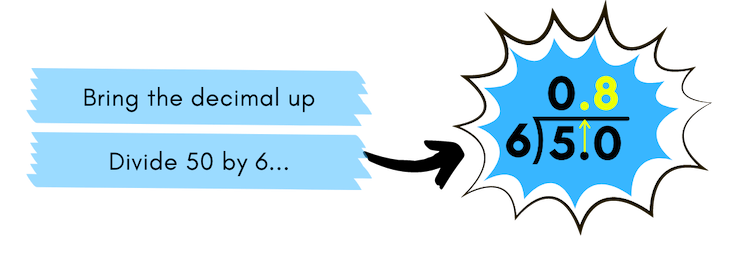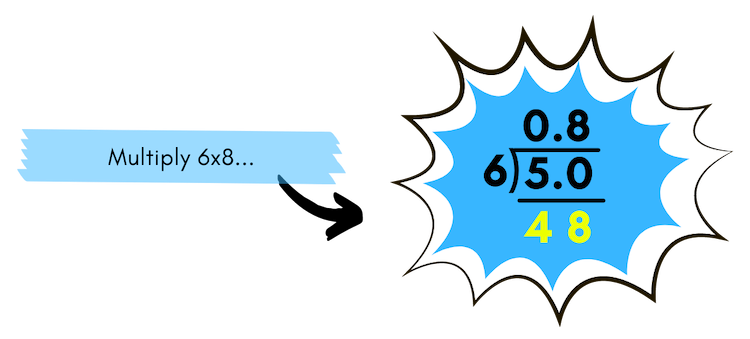#### Step 3: Address the remainder

Since we have a remainder of 2, we need to add another 0 to the end of 5.0 and bring the 0 down.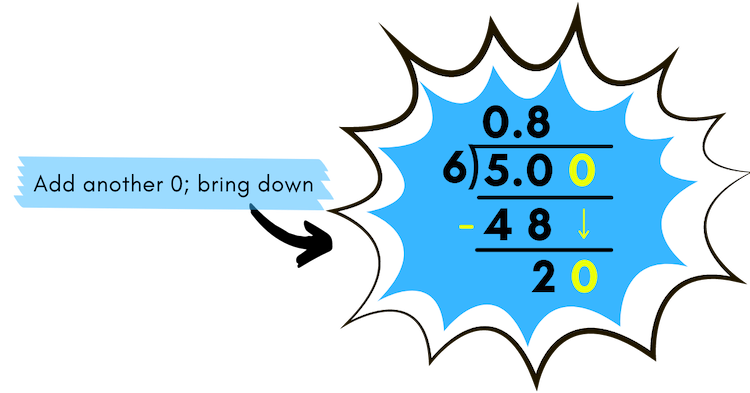Now ask yourself, how many times does 6 go into 20? 3 is correct, since 3 x 8 = 18, which is as close as you can get to 20 without going over.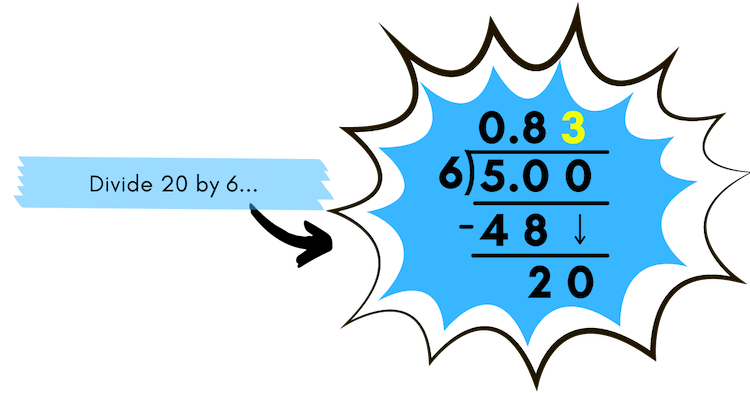#### Step Four: Identify the repeating remainder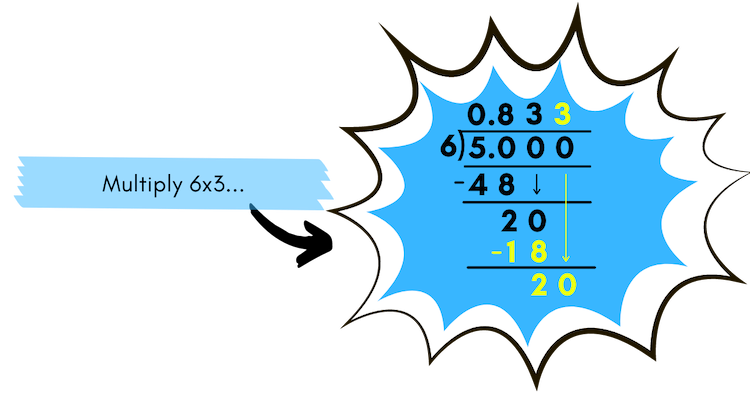You might notice a pattern forming. You are right!

You will continually get a remainder of 2, and instead of writing 3 over and over again, we place a bar over the number(s) that are repeating.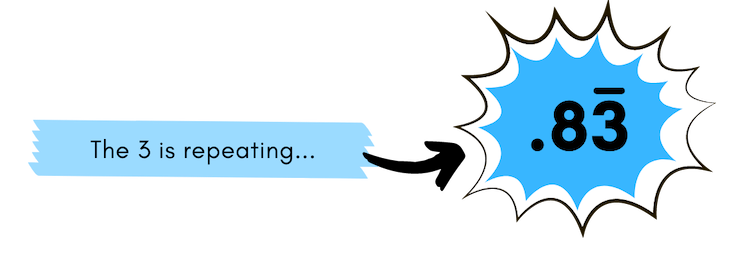In this case, only the 3 is repeating, so we write our final answer as (above).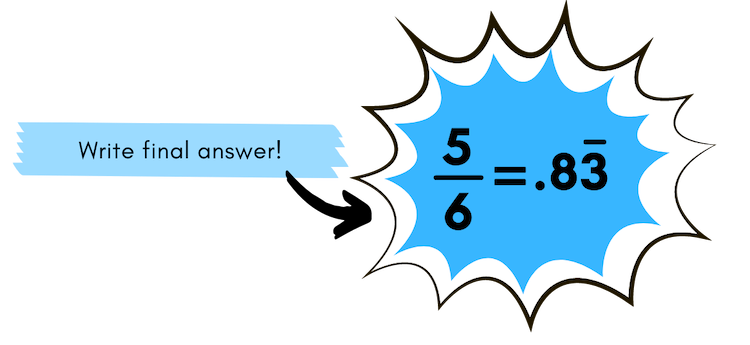This means that ⅚ = .83. This makes sense since ⅚ is close to one whole, and . 83 is also close to one whole.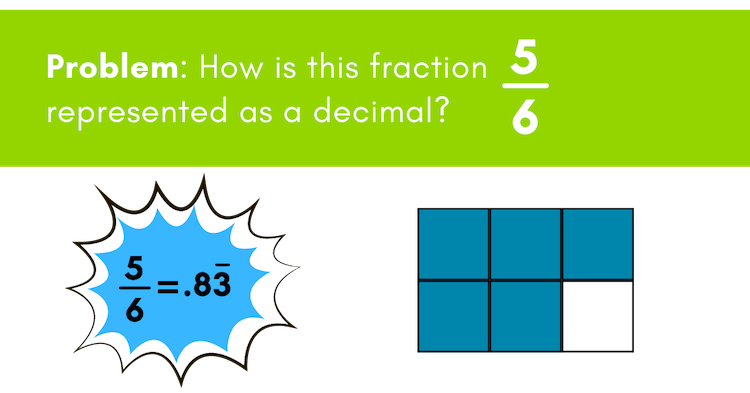Congratulations! You did it. Wondering how to convert decimals into fractions? We’ve got you covered in this related post.

For more math resources, check out our one on one math tutoring opportunitiesVirginia started with iD Tech at the University of Denver in 2015 and has loved every minute since then! A former teacher by trade, she has a master's in education and loves working to embolden the next generation through STEM. Outside the office, you can usually find her reading a good book, struggling on a yoga mat, or exploring the Rocky Mountains.

Featured Posts

Categories

Authors